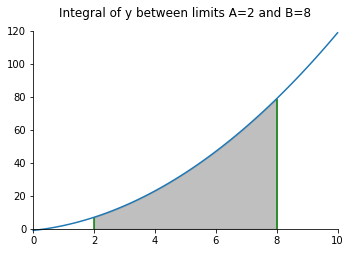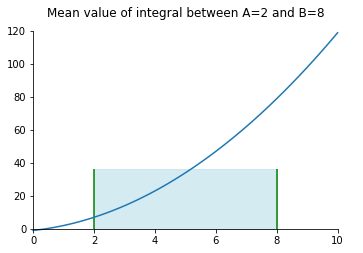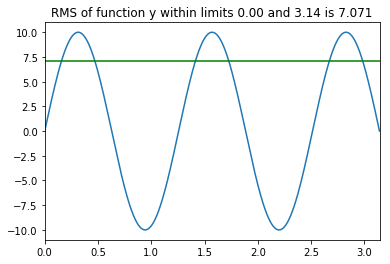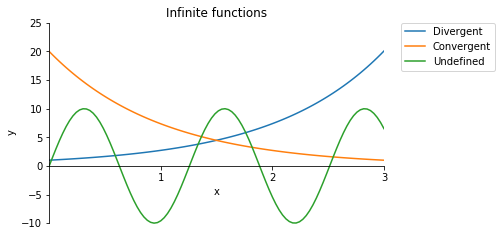Notebook

# Maths

## Integrals

This chapter will show the some basic integration definitions that are often used when dealing with functions or signals.

In :
% run ./maths_functions.py


#### Integration

For a function f(x), the integral of f(x) with limits A and B, is the total area between the function and the y=0 axis.

$A=\int_{A}^{B} f({x}) dx = F({x})$

The length of the function's line between those limits is $L=\int_{s_0}^{s_2} \sqrt{1 + \frac{dy^2}{dx^2}} dx$

For $y=f(t)$ and $x=g(t)$, $L=\int_{s_0}^{s_2} \sqrt{\frac{dy^2}{dt^2} + \frac{dx^2}{dt^2}} dt$

In :
#Define range for x (start, end, number of steps)
X = np.linspace(0,10,100)

#Define function y(x)
def y_1(x):
return x*x+2*x-1

#Define integration limits A and B
A = 2
B = 8

In :
finite_integral_plot(X,y_1,A,B)#### Mean Value of a Signal

The mean value of a function ${y} = f({x})$ is $\bar{y}$ such that for x-limits A and B, the area $(B-A).\bar{y} = \int_A^B y dx$

$\bar{y} = \frac{1}{B-A} \int_{A}^{B} f({t}) dt$

As can be seen in the graph below, a square with height of the mean value and width of the integration limits has the same area as the area under the curve for those limits.

In :
mean_value_example(X,y_1,A,B)#### RMS Value of a Signal

The Root-Mean-Square value of a function y is $\bar{y}_{rms} = \sqrt{\frac{1}{B-A} \int_{A}^{B} f({t})^2 dt}$

Unlike the mean value, the RMS value is always equal to or greater than zero. It is often used to describe average power dissipation or for power calculaltions involving alternating current.

In :
def y_2(x):
return 10*np.sin(5*x)

In :
A = 0
B = np.pi

In :
rms_example(A,B,y_2)#### Infinite Limits of a Sequence

The limit of a sequence is the value to which the function 'tends to'.

• Divergent - undefined/infinite, function tends towards $\infty$ or $-\infty$
• Convergent - defined/finite, function tends towards a finite value
• Undefined - function oscillates and does not tend to any value
In :
X = np.linspace(0,3,100)
Y = np.exp(X)
Y2 = 20/np.exp(X)
Y3 = 10*np.sin(5*X)

In :
inf_example(X,Y,Y2,Y3)